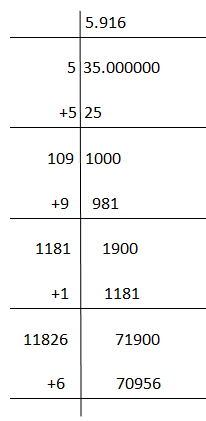Jet Set Go! All about Aeroplanes Jet Set Go! All about Aeroplanes

# Find Square Root

Here, we will learn to find the square root of different numbers, in the fastest way. Although the easiest way to find it is by using a calculator. But, students should learn to solve the mathematical problems on their own instead of using calculators. This practice will help them to build their confidence and develop problem-solving skills. Therefore, we will learn here how to find the square root of numbers without using calculators.

Basically, there are two ways by which we can find the root: Prime factorisation and long division method. We will learn here both the methods to understand how to get the square roots. Also, learn here tricks for solving square roots.

## Calculation of Square Roots

The square root of a number is a value which when multiplied by itself gives the original number. For example, the square root of 4 is 2 and when 2 is multiplied by 2 it is again equal to 4.

The symbol used to represent square root is ‘√’. This symbol is called radical and the number under the radical symbol is said to be radicand.

The number present under the root can be a perfect square or imperfect square. For example, 9 is a perfect square, 3 x 3 = 32 = 9 but 5 is an imperfect square.

When we find the square root of a perfect square it is a natural number but the square root of an imperfect square is a fraction.

Now let us find out how to calculate the square root of different numbers.

### Using Prime Factorisation

Let us see some examples to find the square root using prime factorisation.

1. Square root of 400.

By prime factorisation, we get;

400 = 2 x 2 x 2 x 2 x 5 x 5

Now pairing the common numbers in a pair of two, we see here three pairs.

Two pairs of 2 and one pair of 5

Therefore,

√400 = √(2 x 2 x 2 x 2 x 5 x 5)

Taking out the numbers in pairs.

√400 = 2 x 2 x 5 = 20

Hence, the square root of 400 is 20.

2. Square root of 121

By prime factorisation, we get:

121 = 11 x 11

We can see here there is only one pair of 11.

So, by taking the square root;

√121 = √(11 x 11)

Take out the number in pairs of two.

√121 = 11

Hence, square root of 121 is 11

3. Square root of 169

By prime factorisation, we know:

169 = 13 x 13

As we can see, there is one pair of 13.

Now, by taking the square root on both sides.

√169 = √(13 x 13)

Take out the number under root in pairs.

√169 = 13

Hence, the square root of 169 is 13.

### Using Long Division Method

This method is specially used to find the square root of large numbers and the imperfect squares. Let us see some examples based on this method to find the square root.

1. Find the square root of 35.

Since 35 is an imperfect square, therefore we can use prime factorisation.In the same way, we can find the square root of some more imperfect squares such as 2, 3, 5, 6, 7, 8, 10, etc. Below is the list of the square root of imperfect squares.

 Imperfect square Square root 2 1.414 3 1.732 5 2.236 6 2.449 7 2.646 8 2.828 10 3.162 11 3.317 12 3.464 13 3.606 14 3.742 15 3.873

Related Articles

### Video LessonQuiz on Square root